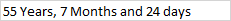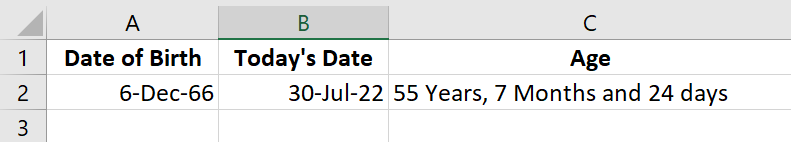# How to Subtract a Date From Today in Excel

Even though Microsoft Excel spreadsheet software was created for numerical calculations, we often have dates within our data, and we need to calculate elapsed days, weeks, months, years, or sometimes just working days.

Fortunately, Excel has you covered pretty well.

All of these operations are easily possible, and we can calculate anything related to dates – be in working days, months, or years, using a plethora of Excel functions and a number of ways.

## How Are Dates Stored in Microsoft Excel

When you type a date in Excel, it appears as a Date. In the format section, you will even see it as a Date Format. But strangely, Microsoft Excel has no special way of determining dates as a Date data type. So a Date value is stored as a number in Excel. This trend was started by Lotus 1-2-3, the spreadsheet that predates Microsoft Excel.

So when you type a date in Excel, it is converted to a number – a serial number to be exact. This serial number starts from 1-March-1900. This first date was numbered 1, and then subsequent dates were calculated by adding days to this number.

If you don’t believe me, simply type a date and then change its type from Date to General. You’ll see a large number appear instead of the date. Something like 44762. The serial number you get might be higher or lower than this number, depending on the date you have keyed in.

While you may be puzzled by the fact that dates are being stored as serial numbers in Excel, it is in fact a boon for us.

Now we can perform arithmetic with the dates, as they are not really “dates” but rather “numbers”.

Do take note that based on your regional settings, you might see the date pre-formatted as DD/MM/YY or MM/DD/YY.

Further, you might see a two-digit year or a four-digit year. To tweak the way dates appear, you can change the format by picking up a suitable Custom Format.

## How To Find Today’s Date

You can find the current date by using the Excel function TODAY. This is an empty function and does not take any arguments. Simply write =TODAY(), and you will get today’s date.

This makes it easy to make other dates, like tomorrow and yesterday, with the help of the today function.

Today =TODAY()

Tomorrow =TODAY() + 1

Yesterday = TODAY() – 1

Similarly, you can add or subtract any number of days from a starting date by using this method. Do try it out on any given date.

If you want to see the date with time, you can use the equivalent time function of Excel – NOW()

When you use NOW(), you get the date, along with the current time. Both the TODAY() & NOW() are dynamic functions, and every time any other function is evaluated in Excel, the Today & Now functions would get updated too to the latest current date and time.

## How to Subtract a Date from Today

Instead of making future or past dates, you might already have a date, like the date of birth, and now you want to find the age of a person.

This can be achieved by subtracting the second date from the first date. So in our case, we will subtract the date of birth from Today’s date.

As an example, if the cell A2 contains the date of birth and the cell B2 contains Today’s date, then we can compute the difference between the two dates in cell C2 with the following formula:

=B2 – A2

You can try this on a blank Excel sheet.

The above formula will result in a number being shown in cell C2. The number obtained is the number of days difference between the two dates. Keep in mind that whenever you use this formula, you may receive a positive value or a negative value, depending on how you subtract dates.

If you subtract the earlier date from the current date, you will get a positive number, and when subtracting the current date from a previous date will give you a negative number.

## How to Find the Age of a person in Excel

Calculating the number of days elapsed between 2 dates and getting a number is good, but if you want to calculate the Age of a person, having the difference in days isn’t much useful. We now need to convert the elapsed days into number of years, the number of months and the number of Days.

Calculating these three things manually can require a lot of calculations, using the Day functions of Excel. We can use many different ways to calculate the difference in Excel dates.

There’s an absolute gem of a function called the DATEDIF function in Excel, which is actually meant for subtracting dates, and find the difference between dates in elapsed days. It can tell you the difference in Years, Months & Days, depending on the third argument provided within the function.

I generally prefer this easy way to find the difference in days between specific dates. Datedif takes the following arguments.

DATEDIF(start_date, end_date, unit)

In this function, the first argument is the start date. The second argument is the end date or the due date. The unit can be anything from the list below.

“Y” returns the number of complete years in the period.

“M” returns the number of complete months in the period.

“D” returns the number of days in the period.

“MD” returns the difference between the days in start_date and end_date. The months and years of the dates are ignored.

“YM” returns the difference between the months in start_date and end_date. The days and years of the dates are ignored.

“YD” returns the difference between the days of start_date and end_date. The years of the dates are ignored. Only the difference between the dates without the years, is calculated.

So to calculate the complete years between any 2 selected dates, we can use the DATEDIF function in the third blank cell. The date_of_birth cell references the start date.

The todays_date cell references the cell where we have written =TODAY() to get Today’s date.

=DATEDIF(date_of_Birth, todays_date, “Y”)

To calculate the complete months after the years, we can use:

=DATEDIF(date_of_Birth, todays_date, “YM”)

And to calculate the complete days between 2 dates ignoring months and years, we can use:

=DATEDIF(date_of_Birth, todays_date, “MD”)

And to calculate the exact age, we can then combine the 3 functions, using the CONCATENATE Method, or using the & operator.

In the simple formula below, we calculate the complete age of a person born on 6-December-1966, and Today’s date being 30-July-2022.

You can copy and paste this formula and then hit the Enter key.

=DATEDIF(date_of_birth, todays_date, “Y”) & ” Years, ” & DATEDIF(date_of_birth, todays_date, “YM”) & ” Months and ” & DATEDIF(date_of_birth, todays_date, “MD”) & ” days”

For the formula result, you will see## How to Separate Day, Month & Year from any Date

There are several Date & Time Functions in Excel, that can be very clinical and separate out some components of the date, like the days, months or years, as desired.

To get the Day, we use the Day Function:

=DAY( TODAY() ) gives the date like 30

=MONTH( TODAY() ) gives the month as a number from 1 to 12.

As an example, the month function can be helpful in finding out the month of the invoice date. If you have multiple dates in a table, you can simply pull the fill handle and get Excel to calculate the months of all dates.

=YEAR( TODAY() ) gives the year of the year, like 1966 or 2022. Future values of the Year function will yield 2023, 2024 etc.

## Calculating Working Days Between 2 Dates

The DateDif function calculates the number of days between two days. While it is suitable for age, sometimes you want to calculate working days, excluding the weekends.

So you may want to exclude Saturday or Sunday or both, or any other weekday from the calculation and work with only business days as the total number of days elapsed.

In this case, you can use the NETWORKDAYS() function of Microsoft Excel.

Here’s the step-by-step detailed article on how to calculate the difference between two days without the weekends.